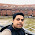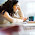## How To Workout Decision Tree Algorithm

Decision Tree Induction is the learning of decision trees from class-labeled training tuples. A decision tree is a flowchart-like tree structure, where each internal node (nonleaf node) denotes a test on an attribute, each branch represents an outcome of the test, and each leaf node (or terminal node) holds a class label. The topmost node in a tree is the root node.

During the late 1970s and early 1980s, J. Ross Quinlan, a researcher in machine learning, developed a decision tree algorithm known as ID3 (Iterative Dichotomiser). This work expanded on earlier work on concept learning systems, described by E. B. Hunt, J. Marin, and P. T. Stone. Quinlan later presented C4.5 (a successor of ID3), which became a benchmark to which newer supervised learning algorithms are often compared. In 1984, a group of statisticians (L. Breiman, J. Friedman, R. Olshen, and C. Stone) published the book Classification and Regression Trees (CART), which described the generation of binary decision trees. ID3 and CART were invented independently of one another at around the same time, yet follow a similar approach for learning decision trees from training tuples. These two cornerstone algorithms spawned a flurry of work on decision tree induction. ID3, C4.5, and CART adopt a greedy (i.e., nonbacktracking) approach in which decision trees are constructed in a top-down recursive divide-and-conquer manner. Most algorithms for decision tree induction also follow such a top-down approach, which tarts with a training set of tuples and their associated class labels. The training set is recursively partitioned into smaller subsets as the tree is being built.

Problem 1:

Iteration 1:

Find gain for class: Play
Calculate the no of yes  and no
No – 5
Yes – 9
Total observation – 14

Entropy([9,5]) = (-9/14 log 9/14 – 5/14 log 5/14)/log 2 = 0.940

Then find the gain for all instance.

EntropyOutlook([2,3],[4,0],[3,2]) = 5/14(-2/5 log 2/5 – 3/5 log 3/5) + 4/14(-4/4 log 4/4) + 5/14(-3/5 log 3/5 – 2/5 log 2/5) /log 2

EntropyTemp([3,1],[4,2],[3,1])/log 2
EntropyHumidity([4,3],[6,1])/log 2
EntropyWindy([2,6],[3,3])/log 2

Therefore
gain(outlook)  = Entropy([9,5]) – EntropyOutlook([2,3],[4,0],[3,2])=0.247
gain(Temp)       = 0.029
gain(humidity)   = 0.152
gain(windy)      = 0.048

Find which is having highest gain and it will be taken as the root node.

So here in overcast all the leaf nodes are yes therefore we don't have a further recursion in that.You can delete the entire row which is having overcast as outlook or else continue with the same table.

Iteration 2:

Now we need to identify what all nodes can come under oulook -> Sunny and outlook -> Rainy.
First look for the case of Sunny. Sort out the master table to a child table which comprises only Sunny entry.

Calculate the no of yes  and no
No - 3
Yes - 2

Entropy([3,2])        = 0.97095

gain(Temp)       = 0.57095
gain(humidity) = 0.97095
gain(windy)       = 0.419973

Find which is having the highest gain
gain(humidity) = 0.97095

So humidity comes under Sunny
New tree becomes

From the above diagram all leaf node is same . Therefore no iteration with in humidity so remaining left out is Rainy.

Iteration 3:

Now we need to identify what all nodes can come under oulook -> Rainy .
Sort out the master table to a child table which comprises only Rainy entry as done in Iteration 2.

Calculate yes and no
No -2
Yes - 3
Entropy([3,2])    = 0.97095

gain(Temp)   = 0.02095
gain(windy) = 0.97095

Highest gain is for windy

therefore it comes under outlook ->rainy .

Now all the leaf is unique so no further iteration.So Temperature will not be taken .

### Algorithm

1. Start
2. Get Input
3. Extract class Label col and find TotalEntropy
4. Associate attr with class label and calc Info gain for each attribute
5. Determine the attribute with highest gain
6. If entropy =0 go to step 7 else go to step 3
7. End

### ID3 to C4.5

Only difference is we will be considering “Split Info” also. So Gain ratio of each attribute will be gain/split info.Other procedures are same.

ex.Split info = info([5,4,5]) = 5 sunny ,4 overcast and 5 rainy like wise.

Reference:

 K.P.Soman,Shyam Diwaker,V.Ajay(2006),Insight into Data Mining Theory And Practice,Prentice-Hall of India Private Limited, New Delhi

1.2.